26 Pure Anonymous Functions
Using pure functions will let us unlock a new level of power in the Wolfram Language, and also let us redo some of the things weve done before in a simpler and more elegant way.
Apply Blur to each image in the list:
 In:=Out=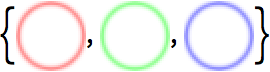But now lets say we want to include the parameter 5 in Blur. How can we do that? The answer is to use a pure function.
Include a parameter by introducing a pure function:
 In:=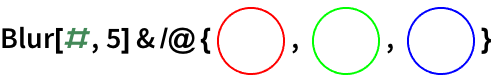Out=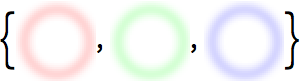The original blur written as a pure function:
 In:=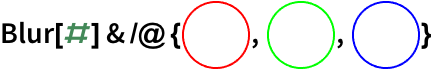Out=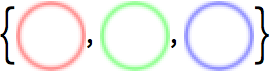The # is a slot into which each element is put. The & says that what comes before it is a pure function.
Heres the equivalent of Blur[#, 5]&/@... expanded out:
 In:=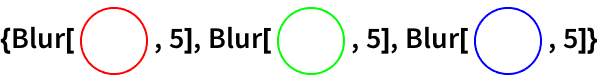Out=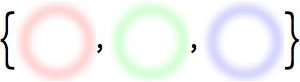In:=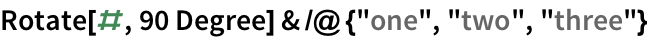Out=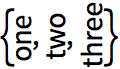Take a string and rotate it different amounts:
 In:=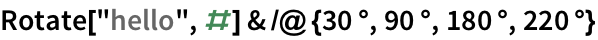Out=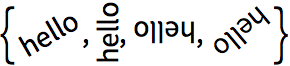In:=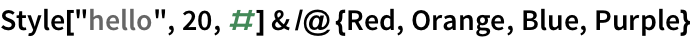Out=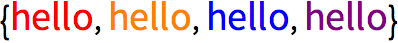In:=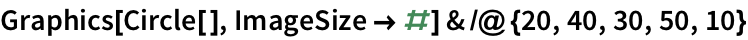Out=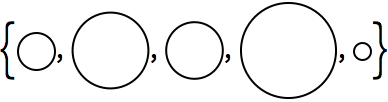In:=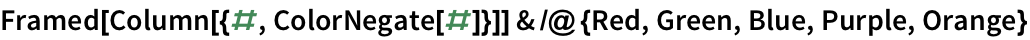Out=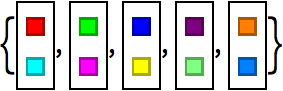In:=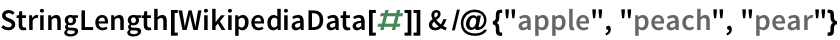Out=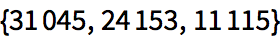Pair topics with results:
 In:=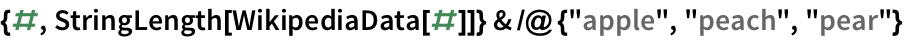Out=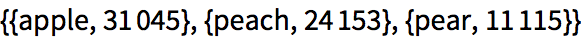In:=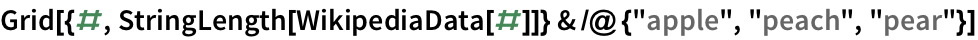Out=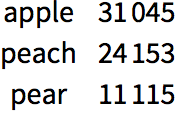In:=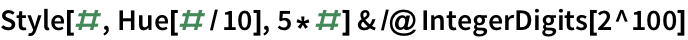Out=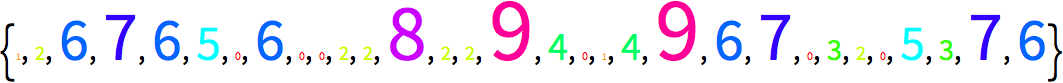Heres what the pure function would do if mapped over {6, 8, 9}:
 In:=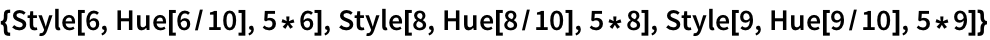Out=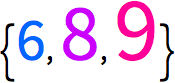Now that weve seen some examples of pure functions in action, lets look more abstractly at whats going on.
This maps an abstract pure function over a list:
 In:=Out=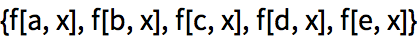Heres the minimal example:
 In:=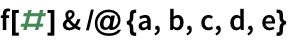Out=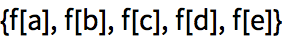Its equivalent to:
 In:=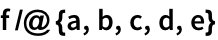Out=We can put slots wherever we want in the pure function, as many times as we want. All the slots will get filled with whatever the pure function is applied to.
Apply a slightly more complicated pure function:
 In:=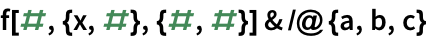Out=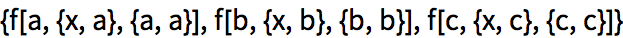In:=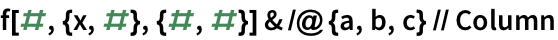Out=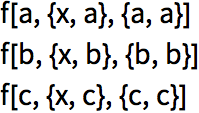But the point is that we can also replace f with a pure function. Then whatever we apply this to will be used to fill the slot in the pure function.
Apply a pure function to x, so the # slot gets filled with x:
 In:=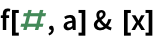Out=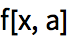An equivalent form, written with @ instead of [...]:
 In:=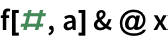Out=So now we can see what /@ is doing: its just applying the pure function to each element in the list.
 In:=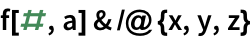Out=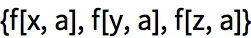The same thing, written out more explicitly:
 In:=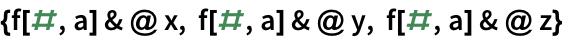Out=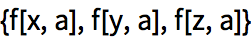Why is this useful? First of all, because its the foundation for all the things pure functions do with /@. But its actually also often useful on its own, for example as a way to avoid having to repeat things.
Heres an example of a pure function involving three occurrences of #.
Apply a pure function to Blend[{Red, Yellow}]:
 In:=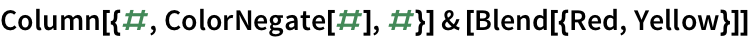Out=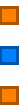In:=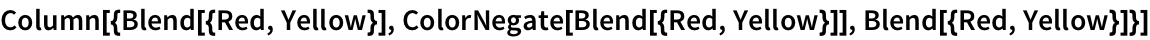Out=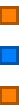In the Wolfram Language, a pure function works just like anything else. On its own, though, it doesnt do anything.
Enter a pure function on its own and itll come back unchanged:
 In:=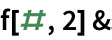Out=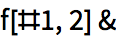Give it to the function Map (/@), though, and itll be used to do a computation.
Map uses the pure function to do a computation:
 In:=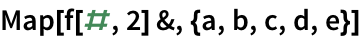Out=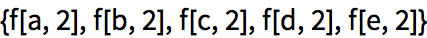Over the course of the next few sections, well see more and more uses of pure functions.
 code& a pure function # slot in a pure function
26.1Use Range and a pure function to create a list of the first 20 squares. »
Expected output:
 Out[]=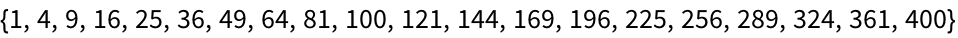Answer & check your solution
26.2Make a list of the result of blending yellow, green and blue with red. »
Expected output:
 Out[]=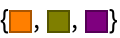Answer & check your solution
26.3Generate a list of framed columns containing the uppercase and lowercase versions of each letter of the alphabet. »
Expected output:
 Out[]=Answer & check your solution
26.4Make a list of letters of the alphabet, in random colors, with frames having random background colors. »
Sample expected output:
 Out[]=Answer & check your solution
26.5Make a table of G5 countries, together with their flags, and arrange the result in a fully framed grid. »
Expected output:
 Out[]=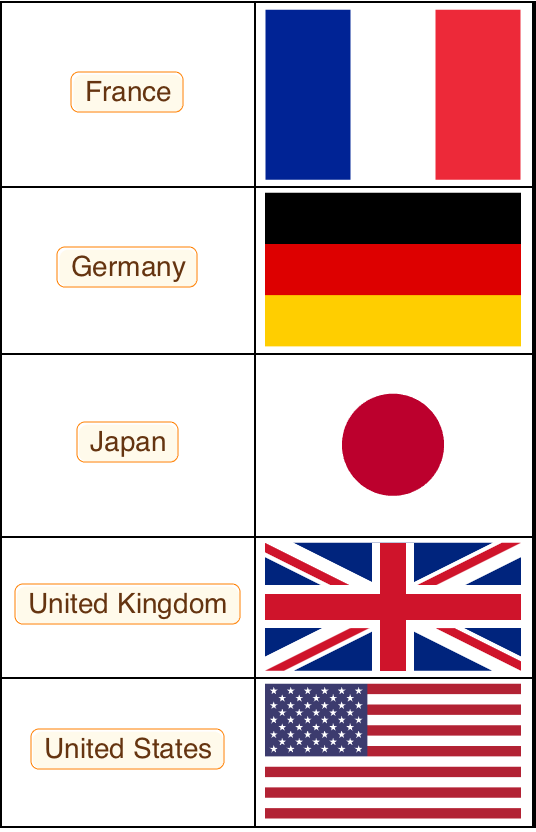Answer & check your solution
26.6Make a list of word clouds for the Wikipedia articles about apple, peach and pear. »
Sample expected output:
 Out[]=Answer & check your solution
26.7Make a list of histograms of the word lengths in Wikipedia articles on apple, peach and pear. »
Sample expected output:
 Out[]=Answer & check your solution
Expected output:
 Out[]=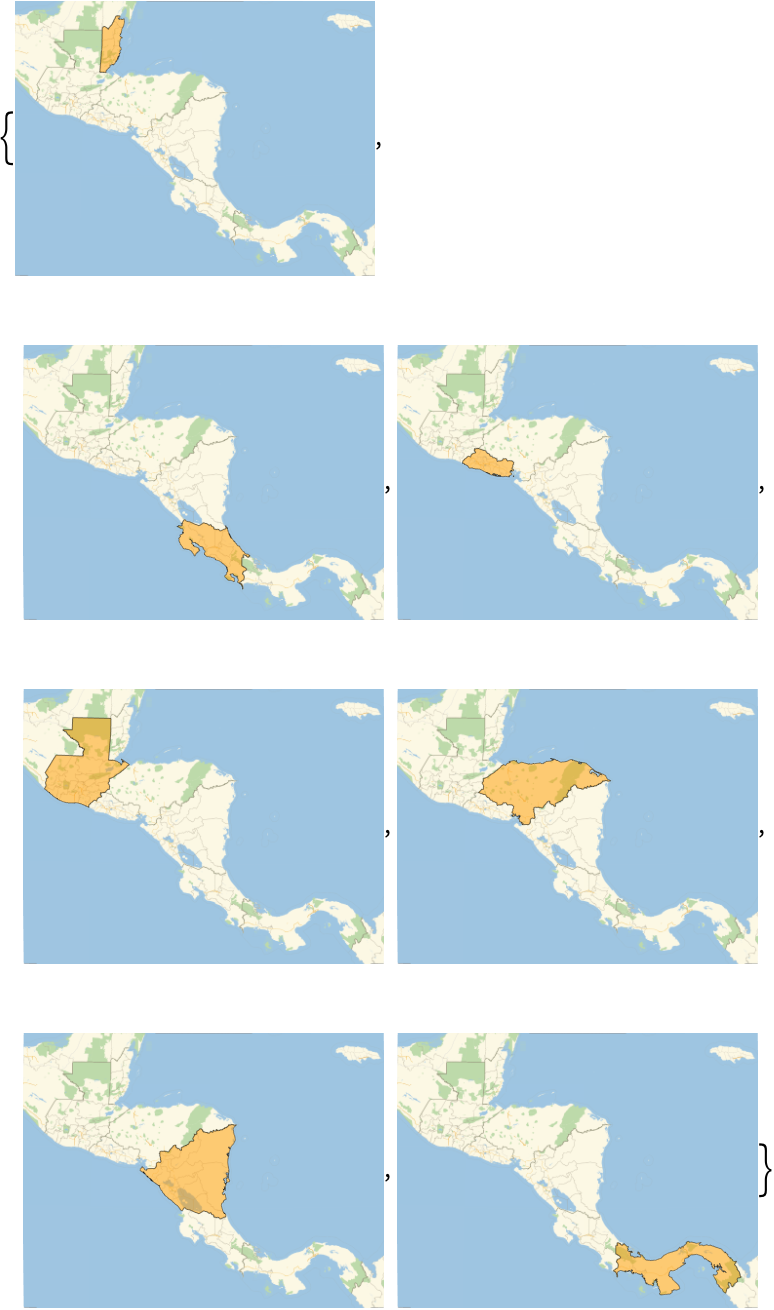Answer & check your solution
+26.1Give a simpler form for (#^2+1&)/@Range»
Expected output:
 Out[]=Answer & check your solution
Why are they called pure functions?
Because all they do is serve as functions that can be applied to arguments. Theyre also sometimes called anonymous functions, because, unlike say Blur, theyre not referred to by a name. Here Im calling them pure anonymous functions to communicate both meanings.
Why does one need the &?
The & (ampersand) indicates that what comes before it is the body of a pure function, not the name of a function. f/@{1, 2, 3} gives {f, f, f}, but f&/@{1, 2, 3} gives {f, f, f}.
What is f[#, 1]& interpreted as?
Function[f[#, 1]]. The Function here is sometimes called the function function.